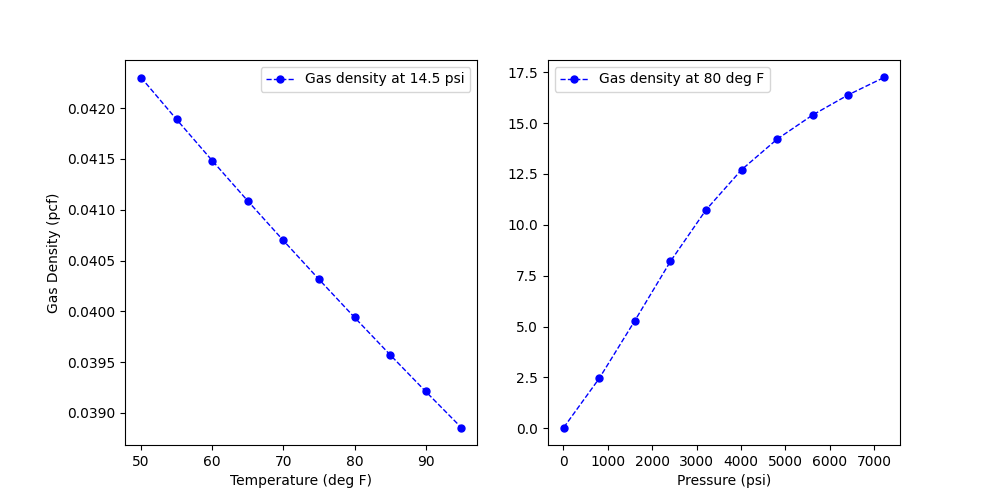# Calculation of gas density

Gas density is calculated using real gas equations from input pressure, temperature and specific gravity of gas. The compressibility factor is calculated explicitly (Beggs and Brill - 1973) for the pressure and temperature combinations. The plot shows the variation of the gas density for methane gas (SG = 0.55) with varying temperature and pressure.```import os

import matplotlib.pyplot as plt
import pandas as pd

from indsl.oil_and_gas.gas_density_calcs import calculate_gas_density as cd

# pressure and temperature series define
base_path = "" if __name__ == "__main__" else os.path.dirname(__file__)
sg = pd.Series([0.5534])
fig, ax = plt.subplots(1, 2, figsize=[10, 5])

ax.plot(
data["T_var (F)"],
cd(data["P_con (psi)"], data["T_var (F)"], sg),
color="blue",
linestyle="dashed",
linewidth=1,
markersize=10,
marker=".",
label="Gas density at " + str(data["P_con (psi)"]) + " psi",
)

ax.set_xlabel("Temperature (deg F)")
ax.set_ylabel("Gas Density (pcf)")
ax.legend()

ax.plot(
data["P_var (psi)"],
cd(data["P_var (psi)"], data["T_con (F)"], sg),
color="blue",
linestyle="dashed",
linewidth=1,
markersize=10,
marker=".",
label="Gas density at " + str(data["T_con (F)"]) + " deg F",
)
ax.set_xlabel("Pressure (psi)")
ax.legend()

plt.show()
```

Total running time of the script: ( 0 minutes 0.385 seconds)

Gallery generated by Sphinx-Gallery